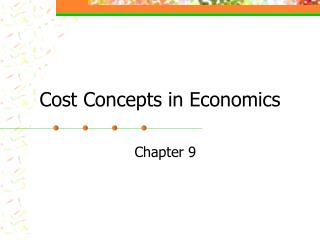# Cost Concepts in Economics - PowerPoint PPT PresentationDownload PresentationCost Concepts in Economics

Cost Concepts in Economics
Download Presentation## Cost Concepts in Economics

- - - - - - - - - - - - - - - - - - - - - - - - - - - E N D - - - - - - - - - - - - - - - - - - - - - - - - - - -
##### Presentation Transcript

1. Cost Concepts in Economics Chapter 9

2. Cost Classification • Fixed or variable • Cash or non-cash • Accounting expense or not • Opportunity costs

3. Opportunity Costs • Not an accounting expense • Every input has an alternative use • OC is the value of product not produced because input was used for another purpose • OC is income that would have been received if input had been used in its most profitable alternative use

4. Opportunity Costs • Not an accounting expense • Every input has an alternative use • OC is the value of product not produced because input was used for another purpose • OC is income that would have been received if input had been used in its most profitable alternative use

5. Short-run and Long-run Costs • Short-run = time period when one or more inputs are fixed • Long-run = time period when all inputs can be changed (none are fixed)

6. Fixed Costs • Costs associated with owning a fixed input or resource • Do not change as level of production changes • Incurred even if not input is used • Not under control of the manager in the short-run • Present in the short-run only

7. DIRTI 5 • Depreciation =

8. DIRTI 5 • Depreciation = • Interest =

9. DIRTI 5 • Depreciation = • Interest = • Repairs • Taxes • Insurance

10. DITI 4 • Depreciation = • Interest = • Taxes • Insurance

11. Example of Fixed Costs • Purchase a pickup for \$20,000 • Salvage value of \$5,000 • Useful life of 5 years • Interest rate of 10% • Taxes are \$25 / year • Insurance is \$1,000 / year

12. Variable Costs • Those costs that the manager has control over in a given period of time • Can be increased or decreased at the manager’s discretion • Feed, seed, fertilizer, etc

13. Total and Average Costs • Total costs = TVC + TFC • Average Variable Costs (AVC) = TVC / Output level • Average Fixed Costs (AFC) = TFC / Output level • Average Total Costs (ATC) = TC / Output level = AVC + AFC

14. Marginal Costs • Cost associated with a change in the output • What did it cost for the last unit of increased output? • MC =

15. Typical Total Cost Curves \$ TC TVC TFC Output

16. Average and MarginalCost Curves \$ ATC MC AVC AFC Output

17. Stocking Rate Problem TFC = \$4000VC = \$395 / steer Selling price = \$70 / cwt

18. Stocking Rate Problem TFC = \$4000VC = \$395 / steer Selling price = \$70 / cwt

19. Production Rules Long-run • SP > ATC • Produce where MR=MC • SP < ATC • Do not produce Short-run • SP > ATC • Produce where MR=MC • ATC > SP > AVC • Making contribution to FC • Produce where MR=MC • SP < AVC • Do not produce

20. Cost Concepts in EconomicsSummary • Cost Classification • Opportunity costs • Fixed, Variable, and Marginal costs • DITI 4 • Average total, fixed, and variable costs • Marginal costs • Cost Curves • Production rules

21. Cost Concepts in Economics Questions?